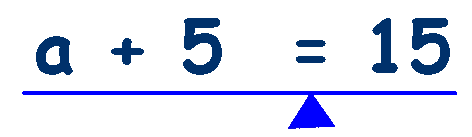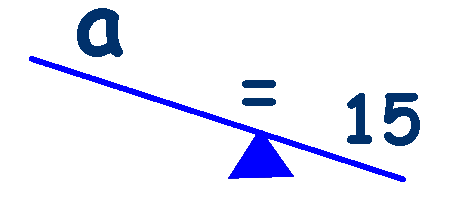# Algebra - Balancing Equations

## 'Do the same to both sides to keep the equation balanced'

In the past, algebraic equations have been represented by a set of weighing scales. This analogy worked well but nowadays children don't know what a set of weighing scales looks like they now have a set of digital scales in the kitchen. I use a seesaw, they all know what a seesaw is from playing on them in the playground, they are easy to draw and you can easily model one by using a ruler and pencil on a table.

The key thing to understand is that an equation has to balance - each side of the equals sign must have the same value for it to balance.

They can relate to having a heavier person on the other end of the seesaw and being stuck up high in the air. Think about an adult on one end and maybe adding friends to their end to balance the seesaw. If their combined weight equals that of the adult then the seesaw would be balanced. Too many children and the adult would left high in the air.

I draw a seesaw and have them imagine what would happen with different combinations of their friends, relatives, dogs, cats, elephants, mice, ants etc on either end - make it crazy and they will remember it. Now move on to numbers and do the same.

Next, I begin to write equations on paper, something simple to start with, say 10 + 5 = 15 and I draw a seesaw beneath the equation with the pivot below the equals sign.Both of these equations are balanced - What is the value of 'a' ?

Hopefully they will see that the equations are the same and that a = 10.

Now just look at this equationWhat would happen if we took 5 away from the left side of the equals sign?

It would be unbalanced, with 10 on one side and 15 on the other.Now what would happen if we took 5 away from the right side of the equals sign?The equation is now balanced again.

We took the same away from both sides and the equation remained balanced

Now just look at this equationWhat would happen if we took 5 away from the left side of the equals sign?

It would be unbalanced, with 'a' on one side and 15 on the other.Now what would happen if we took 5 away from the right side of the equals sign?The equation is now balanced again.

We took the same away from both sides and the equation remained balanced
and in the process we discovered that a = 10

## 'Do the same to both sides to keep the equation balanced'

Try this Basic Equations worksheet created by children from Bayswater School

##Rearranging Equations______________________________________________________